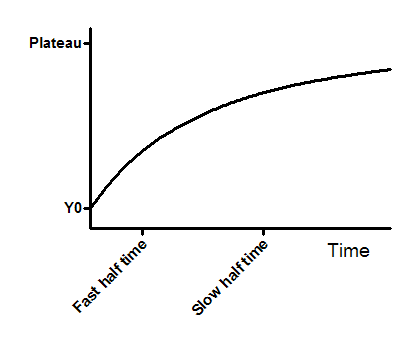## Please enable JavaScript to view this site.

 Equation: Two phase association

## Introduction

An exponential decay equation models many chemical and biological processes. It is used whenever the rate at which something happens is proportional to the amount which is left.

A two-phase model is used when the outcome you measure is the result of the sum of a fast and slow exponential decay.

## Entering data

Create an XY data table. Enter time into X, and response (binding, concentration ..) into Y. If you have several experimental conditions, place the first into column A, the second into column B, etc.

After entering data, click Analyze, choose nonlinear regression, choose the panel of exponential equations, and choose Two phase association.

## Consider constraining Plateau to a constant value of zero

If you have subtracted off any background signal, then you know the curve has to plateau at Y=0. In this case, you should constrain the parameter Plateau to be a constant value equal to zero. To do this, go to the Constrain tab of the nonlinear regression dialog, set the drop down next to Plateau to "Constant equal to" and enter the value 0.0.

## Model

SpanFast=(Plateau-Y0)*PercentFast*.01

SpanSlow=(Plateau-Y0)*(100-PercentFast)*.01

Y=Y0+ SpanFast*(1-exp(-KFast*X)) + SpanSlow*(1-exp(-KSlow*X))Y0 is the Y value when X (time) is zero. It is expressed in the same units as Y,

Plateau is the Y value at infinite times, expressed in the same units as Y.

Kfast and Kslow are the two rate constant, expressed in reciprocal of the X axis time units. If X is in minutes, then K is expressed in inverse minutes.

TauFast and TauSlow are the two time constants, expressed in the same units as the X axis. They are computed as the reciprocals of the rate constants.

Half-time (fast) and Half-time (slow) are in the time units of the X axis. They are computed as ln(2)/K.

PercentFast is the fraction of the span (from Y0 to Plateau) accounted for by the faster of the two components.